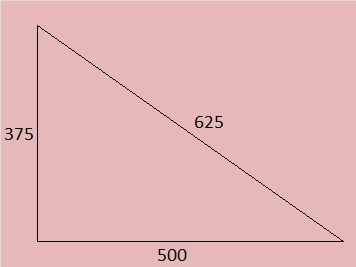# 625 Where did all those Pythagorean triples come from?625 is the hypotenuse of FOUR Pythagorean triples. Where did all those Pythagorean triples come from?

They come from 4 of the 5 factors of 625, all of which are powers of 5, a prime number of the form (4n + 1).

5 is the hypotenuse of the primitive Pythagorean triple 3-4-5 that was calculated from (2^2) – (1^2); 2(2)(1); (2^2) + (1^2).

25 is the hypotenuse of 5 times that triple plus it has a primitive of its own:

• 15-20-25 which is 5 times 3-4-5
• 7-24-25 calculated from (4^2) – (3^2); 2(4)(3); (4^2) + (3^2)

125 is the hypotenuse of 5 times 25’s two triples plus it has a primitive of its own:

• 75-100-125 which is 5 times 15-20-25 or 25 times 3-4-5
• 35-120-125 which is 5 times 7-24-25
• 44-117-125 calculated from 2(11)(2); (11^2) – (2^2); (11^2) + (2^2)

625 is the hypotenuse of 5 times 125’s three triples plus it has a primitive of its own:

• 375-500-625 which is 5 times 75-100-125, or 25 times 15-20-25, or 125 times 3-4-5
• 175-600-625 which is 5 times 35-120-125, or 25 times 7-24-25
• 220-585-625 which is 5 times 44-117-125
• 336-527-625 calculated from 2(24)(7); (24^2) – (7^2); (24^2) + (7^2)

—————————————————————————————————625 is also the short leg of FOUR Pythagorean triples. Where did all those Pythagorean triples come from?

They come from 4 of the 5 factors of 625, all of which are odd. (Every odd number greater than one is the short leg of at least one Pythagorean triple.)

5 is the short leg of the primitive Pythagorean triple 5-12-13. Notice that 12 + 13 = 25 which is 5^2.

25 is the short leg of 5 times that triple plus it has a primitive of its own:

• 25-60-65 which is 5 times 5-12-13
• 25-312-313; Notice that 312 + 313 = 625 = (25^2)

125 is the short leg of 5 times 25’s two triples plus it has a primitive of its own:

• 125-300-325 which is 5 times 25 -60-65 or 25 times 5-12-13
• 125-1560-1565 which is 5 times 25-312-313
• 125-7812-7813; Notice that 7812 + 7813 = (125^2)

625 is the short leg of 5 times 125’s three triples plus it has a primitive of its own:

• 625-1500-1625 which is 5 times 125-300-325, or 25 times 25-60-65, or 125 times 5-12-13
• 625-7800-7825 which is 5 times 125-1560-1565 or 25 times 25-312-313
• 625-39060-39065 which is 5 times 125-7812-7813
• 625-195312-195313; Notice that 195312 + 195313 = (625^2)

Thus 625 appears in 8 Pythagorean triples, and now you know where they all came from.

—————————————————————————————————

Here are some other fun facts about the number 625:

Look at this pattern:

• (5^2) = 25
• (25^2) = 625
• (625^2) = 390,625
• but (390,625^2) = 152,587,890,625 so one digit discontinued the pattern!

625 is the sum of the seven prime numbers from 73 to 103.

What would happen if we ran the following prime number tests on 625?

(24^2) + (7^2) = 625, and 24 and 7 have no common prime factors. That means that 625’s only possible prime factors less than √625 are 5, 13, and 17. Obviously 625 is divisible by 5 so it isn’t a prime number.

Also note that (20^2) + (15^2) = 625, but 20 and 15 have a common prime factor, 5. The fact that they have a common prime factor means that 625 cannot be a prime number.

—————————————————————————————————

• 625 is a composite number.
• Prime factorization: 625 = 5 x 5 x 5 x 5, which can be written 625 = 5^4
• The exponent in the prime factorization is 4. Adding one, we get (4 + 1) = 5. Therefore 625 has exactly 5 factors.
• Factors of 625: 1, 5, 25, 125, 625
• Factor pairs: 625 = 1 x 625, 5 x 125 or 25 x 25
• 625 is a perfect square and a perfect fourth power. √625 = (√25)(√25) = 25## 2 thoughts on “625 Where did all those Pythagorean triples come from?”

1.Joseph Nebus

625 had fascinated me from childhood — that 25 squared thing is just compelling — although I hadn’t been aware of that sum-of-prime-numbers business.

2.ivasallay

Indeed it is a very good number. Thanks so much for your continued support.

This site uses Akismet to reduce spam. Learn how your comment data is processed.